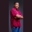Related Tags

swift
set
equality
community creator
communitycreator

# How to check if two sets are equal in SwiftTheodore Kelechukwu Onyejiaku

### Overview

We can check if two sets are equal in Swift by using the equality operator ==. Two sets are equal if both contain the same elements. If they are equal, the operator returns true. Otherwise, it returns false.

### Syntax

set1 == set2
Syntax to check if two sets are equal in Swift

### Parameters

set1: This is a set on the left-hand side of the operator. It is one of the two sets we want to compare.

set2: This is the set on the right-hand side of the operator. It is the other of the two sets that we want to compare.

### Return value

The value returned is a boolean value. If the sets are equal, then a true is returned. Otherwise, a false is returned.

### Code example

// create some sets
let evenNumbers : Set = [10, 2, 6, 8, 4]
let oddNumbers : Set = [11, 5, 7, 3, 9]
let evenNumbers2 : Set = [2, 4, 6, 8, 10]
let first_names : Set = ["john", "jane", "henry", "mark"]
let last_names : Set = ["jane", "john", "henry", "mark"]

// check if equal
print(evenNumbers == oddNumbers)   // false
print(evenNumbers == evenNumbers2) // true
print(first_names == last_names)   // true
Comparing sets in Swift

## Explanation

• Lines 2-6: We create some set instances and initialize them with some values.
• Lines 9-11: We compare the sets to find if they are equal by using the double equality operator. Then we print the results to the console.

RELATED TAGS

swift
set
equality
community creator
communitycreator

CONTRIBUTORTheodore Kelechukwu Onyejiaku
RELATED COURSES

View all Courses

Keep Exploring

Learn in-demand tech skills in half the time